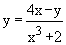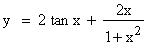Home MonkeyNotes Printable Notes Digital Library Study Guides Study Smart Parents Tips College Planning Test Prep Fun Zone Help / FAQ How to Cite New Title Request

1.4 Classification Of Functions

Analytically represented functions are either Elementary or Non-elementary.

The basic elementary functions are :

1)      Power function :

y = xm , m ÎR

2)      Exponential function :

y = ax , a > 0 but a ¹ 1

3)      Logarithmic function :

y = log ax , a > 0, a ¹ 1 and x > 0

4)      Trigonometric functions :

y = sin x, y = cos x, y = tan x,
y = csc x, y = sec x and y = cot x

5)      Inverse trigonometric functions

y = sin-1 x, y = cos-1x, y = tan-1x,

OR    y = cot-1x, y = cosec-1x, y = sec-1x.

y = arc sin x, y = arc cos x, y = arc tan x

y = arc cot x, y = arc csc x and y = arc sec xYour browser does not support the IFRAME tag.

Note that an elementary function is a function which may be represented by a single formula like y = f (x) where f(x) is made up of basic elementary functions and constants by means of a finite number of operations of addition, subtraction, multiplication, division and taking the function of a function (composite function).

Consider the following examples :

1. y = 1, 2, 3 ........ n [ y = f (n) ] is not elementary as the number of operations that must be performed to get y is not finite but it increases with 'n'.

2. A function 'f ' is defined as

f (x) = x2 if 0 £ x £ 1

= 3x + 1 if 1 £ x £ 2

It is not elementary as it is not represented by a single formula but two formulae.

• Single valued function : For each value of x, suppose there corresponds one and only one value of y, then y is called a singled valued function.

e.g.,, y = 2 logx + 4e2x

• Index